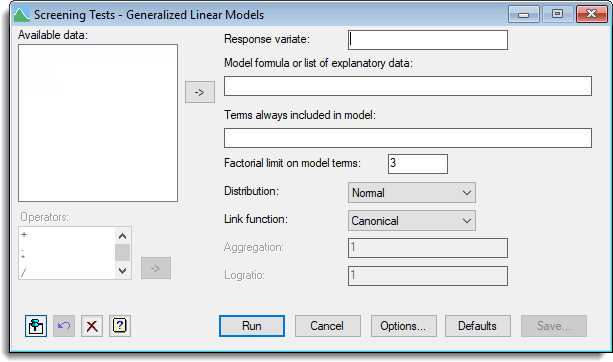1. Home
2. Screening Tests – Generalized Linear Models

# Screening Tests – Generalized Linear Models

Select menu: Stats | Regression Analysis | Screening Tests | Generalized Linear Models

Use this to calculate marginal and conditional tests for all terms in a generalized linear model. It also performs pooled testing of all main effects, of all 2-factor interactions, etc.

Stats | Regression Analysis | Screening Tests | Generalized Linear Models.
2. Fill in the fields as required then click Run.

You can set additional Options then after running, you can save the results by clicking Save.## Available data

This lists data structures appropriate to the current input field. The contents will change as you move from one field to the next. Double-click a name to copy it to the current input field; alternatively, you can type the name directly into the input field.

## Response variate

Specify a response variate containing the data.

## Model formula or list of explanatory data

Specifies the model candidate terms in the fitted model, which may be set using a model formula or using a list of terms separated by commas.

## Terms always included in the model

It is sometimes desirable to include specific terms in every model. Such terms may be specified by in this box, which may be set using a model formula or using a list of terms separated by commas.

## Factorial limit on model terms

You can control the factorial limit on model terms to be generated when you use in model-formula operators like ‘*’.

## Distribution

List of available error distributions. If you select the Binomial distribution then you must supply the Binomial totals in the space provided. Alternatively, if you select the Negative binomial you must supply the Aggregation in the space provided.

Lists the available link functions. If you select the Power link function then you must supply the Exponent in the space provided. Similarly, if you select the Logratio link function then you must supply the Logratio in the space provided.

## Binomial totals

For a Binomial distribution, this specifies a variate which contains the number of units the response was obtained from. This values in this must be greater than or equal to the corresponding values in the Response variate.

## Operators

This provides a quick way of entering operators in the model terms formula. Double-click on the required symbol to copy it to the current input field. You can also type in operators directly. See model formula for a description of each.